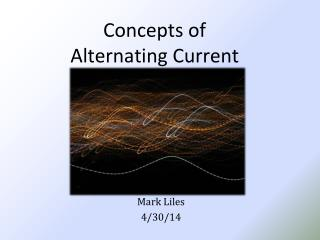# Concepts of Alternating Current - PowerPoint PPT PresentationDownload PresentationConcepts of Alternating Current

Concepts of Alternating Current
Download Presentation## Concepts of Alternating Current

- - - - - - - - - - - - - - - - - - - - - - - - - - - E N D - - - - - - - - - - - - - - - - - - - - - - - - - - -
##### Presentation Transcript

1. Concepts of Alternating Current Mark Liles 4/30/14

2. Learning Objectives • State the differences between AC and DC voltage and current. • State the advantages of AC power transmission over DC power transmission. • State the “left-hand rule” for a conductor • State the relationship between current and magnetism.

3. AC and DC • Alternating current is current which: • Constantly changes in amplitude, • Reverses direction at regular intervals

4. Disadvantage of DC compared to AC • DC voltage must be generated at the level (amplitude or value) required by the load. • Power dissipation needed to “step down” voltages, this is very inefficient • DC system is inefficient when transmitting power over long distances. • I2R loss

5. Disadvantage of DC compared to AC • Unlike DC, AC can be stepped up or down in amplitude by a TRANSFORMER. Power Line Transformer Receptacle Transformer

6. Voltage Waveforms

7. Electromagnetism • Electromagnetism - magnetism developed by a current of electricity. • An electric current always produces some form of magnetism. • The most commonly used means for producing or using electricity involves magnetism.

8. Conductors and Magnetic Fields • If a compass is placed in the vicinity of a current-carrying conductor, the compass needle will align itself at right angles to the conductor.

9. Conductor “left-hand rule” • Left-Hand Rule for a Conductor: If you grasp the conductor in your left hand with the thumb extended in the direction of current flow (- to +), your fingers will point in the direction of the magnetic lines of force

10. Current Flow and Magnetism • The diagrams below show a cross section of a conductor (dot). The behavior of the magnetic fields are shown for • (A) current flowing toward the viewer • (B) current flowing away from the viewer

11. Current Flow and Magnetism • When two adjacent parallel conductors carry current in the same direction, the magnetic fields combine and increase the strength of the field:

12. Current Flow and Magnetism • When two adjacent parallel conductors carry current in opposite directions, the magnetic fields repel each other:

13. Magnetic Field of a Coil • When current is passed through a coil, the magnetic fields link and create a two pole field similar to a magnet.

14. Magnetic Field of a Coil • The strength of a coil’s magnetic field depends on: • Number of turns of wire in the coil • Amount of current flowing in the coil • Ratio of coil with to length • Type of core material

15. Conclusion • Class review: • What are the differences between AC and DC voltage and current? • State one advantage of AC power transmission over DC power transmission. • What is the “left-hand rule” for a conductor? • Summarize the relationship between current and magnetism.